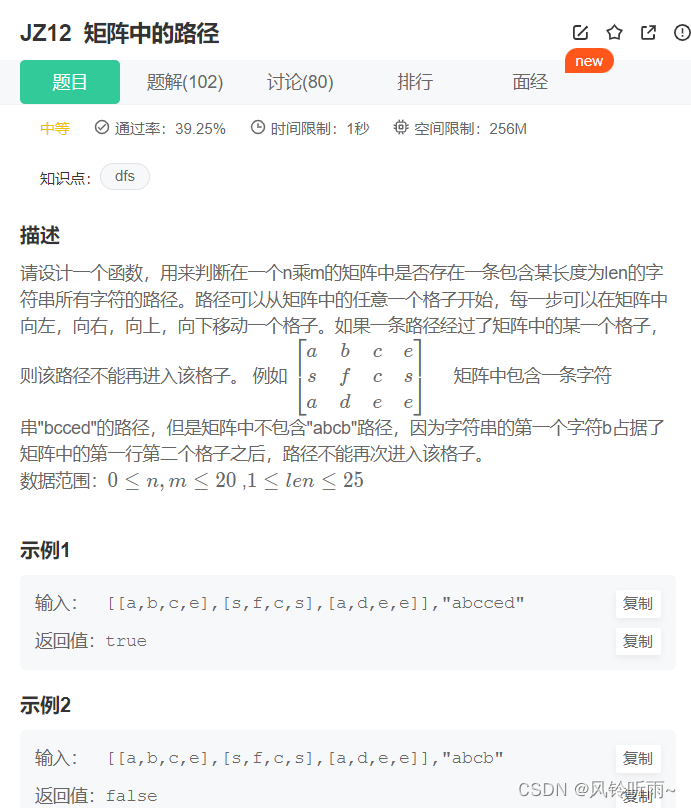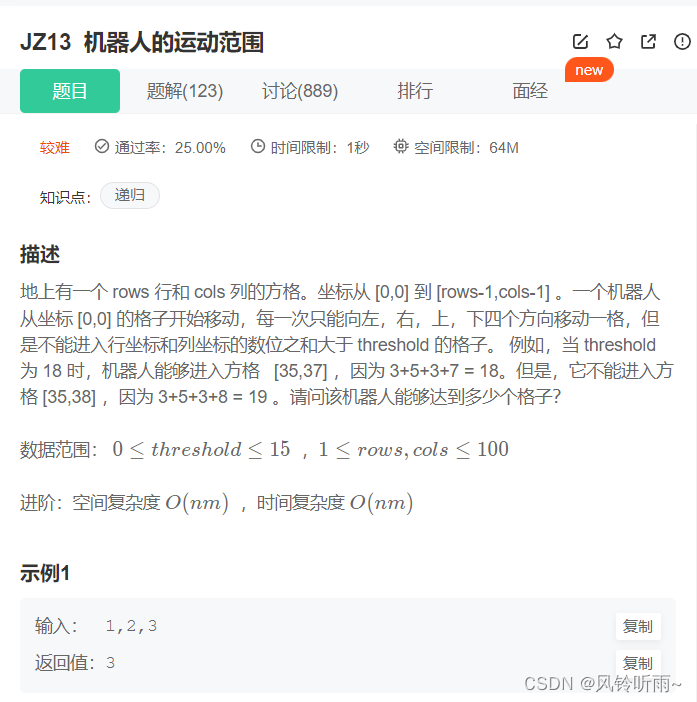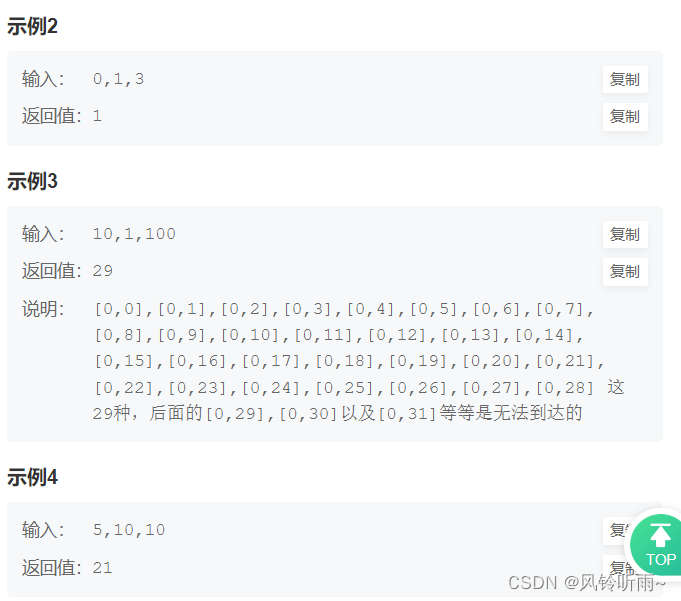> 文档中心 > 【java刷算法】牛客—剑指offer4DFS与BFS两种思路的碰撞，一起来练习吧

# 【java刷算法】牛客—剑指offer4DFS与BFS两种思路的碰撞，一起来练习吧

• 🧛‍♂️个人主页：杯咖啡
• 💡进步是今天的活动，明天的保证！
• ✨目前正在学习：SSM框架,算法刷题
• 👉本文收录专栏 ： java刷算法牛客—剑指offer
• 🙌牛客网，刷算法过面试的神级网站，用牛客你也牛。 👉免费注册和我一起学习刷题👈
• 🐳希望大家多多支持🥰一起进步呀！
• 😎The man who fears losing has already lost.
怕输的人已经输了。 - 《权力的游戏》

# ✨今日二剑

### 文章目录

• ✨今日二剑
• JZ12 矩阵中的路径
• JZ13 机器人的运动范围
• JZ12 矩阵中的路径
• 题目描述
• 思路详解
• 代码与结果
• JZ13 机器人的运动范围
• 题目描述
• 思路详解
• DFS（深度优先搜索）
• BFS（广度优先搜索）
• 代码与结果
• DFS（深度优先搜索）
• BFS（广度优先搜索）
• ✨总结

# JZ12 矩阵中的路径

## 题目描述## 代码与结果

import java.util.*;public class Solution {    /**     * 代码中的类名、方法名、参数名已经指定，请勿修改，直接返回方法规定的值即可     *     *      * @param matrix char字符型二维数组      * @param word string字符串      * @return bool布尔型     */   public boolean hasPath(char[][] matrix, String word) {    char[] words = word.toCharArray();    for (int i = 0; i < matrix.length; i++) { for (int j = 0; j < matrix.length; j++) {     //从[i,j]这个坐标开始查找     if (dfs(matrix, words, i, j, 0))  return true; }    }    return false;} boolean dfs(char[][] matrix, char[] word, int i, int j, int index) {    //边界的判断，如果越界直接返回false。index表示的是查找到字符串word的第几个字符，    //如果这个字符不等于matrix[i][j]，说明验证这个坐标路径是走不通的，直接返回false    if (i >= matrix.length || i < 0 || j >= matrix.length || j < 0 || matrix[i][j] != word[index]) return false;    //如果word的每个字符都查找完了，直接返回true    if (index == word.length - 1) return true;    //把当前坐标的值保存下来，为了在最后复原    char tmp = matrix[i][j];    //然后修改当前坐标的值    matrix[i][j] = '.';    //走递归，沿着当前坐标的上下左右4个方向查找    boolean res = dfs(matrix, word, i + 1, j, index + 1)     || dfs(matrix, word, i - 1, j, index + 1)     || dfs(matrix, word, i, j + 1, index + 1)     || dfs(matrix, word, i, j - 1, index + 1);    //递归之后再把当前的坐标复原    matrix[i][j] = tmp;    return res;}}# JZ13 机器人的运动范围

## 题目描述## 思路详解

### BFS（广度优先搜索）

BFS不是一条道走下去，他会把离他最近的都访问一遍，访问完之后才开始访问第二近的……，直到访问完全部的。
BFS使用最好的一种数据结构就是使用队列，因为队列是先进先出，离他最近的访问完之后加入到队列中，最先入队的也是最先出队的。

## 代码与结果

### DFS（深度优先搜索）

public int movingCount(int threshold, int rows, int cols) {    //临时变量visited记录格子是否被访问过    boolean[][] visited = new boolean[rows][cols];    return dfs(0, 0, rows, cols, threshold, visited);} public int dfs(int i, int j, int rows, int cols, int threshold, boolean[][] visited) {    //i >= rows || j >= cols是边界条件的判断，threshold < sum(i, j)判断当前格子坐标是否    // 满足条件，visited[i][j]判断这个格子是否被访问过    if (i >= rows || j >= cols || threshold < sum(i, j) || visited[i][j]) return 0;    //标注这个格子被访问过    visited[i][j] = true;    //沿着当前格子的右边和下边继续访问    return 1 + dfs(i + 1, j, rows, cols, threshold, visited) +     dfs(i, j + 1, rows, cols, threshold, visited);} //计算两个坐标数字的和private int sum(int i, int j) {    int sum = 0;    //计算坐标i所有数字的和    while (i != 0) { sum += i % 10; i /= 10;    }    //计算坐标j所有数字的和    while (j != 0) { sum += j % 10; j /= 10;    }    return sum;}### BFS（广度优先搜索）

import java.util.LinkedList;import java.util.Queue; public class Solution {    public int movingCount(int threshold, int rows, int cols) { //临时变量visited记录格子是否被访问过 boolean[][] visited = new boolean[rows][cols]; int res = 0; //创建一个队列，保存的是访问到的格子坐标，是个二维数组 Queue<int[]> queue = new LinkedList<>(); //从左上角坐标[0,0]点开始访问，add方法表示把坐标 // 点加入到队列的队尾 queue.add(new int[]{0, 0}); while (queue.size() > 0) {     //这里的poll()函数表示的是移除队列头部元素，因为队列     // 是先进先出，从尾部添加，从头部移除     int[] x = queue.poll();     int i = x, j = x;     //i >= rows || j >= cols是边界条件的判断，threshold < sum(i, j)判断当前格子坐标是否     // 满足条件，visited[i][j]判断这个格子是否被访问过     if (i >= rows || j >= cols || threshold < sum(i, j) || visited[i][j])  continue;     //标注这个格子被访问过     visited[i][j] = true;     res++;     //把当前格子右边格子的坐标加入到队列中     queue.add(new int[]{i + 1, j});     //把当前格子下边格子的坐标加入到队列中     queue.add(new int[]{i, j + 1}); } return res;    }     //计算两个坐标数字的和    private int sum(int i, int j) { int sum = 0; //计算坐标i所有数字的和 while (i != 0) {     sum += i % 10;     i /= 10; } //计算坐标j所有数字的和 while (j != 0) {     sum += j % 10;     j /= 10; } return sum;    }}